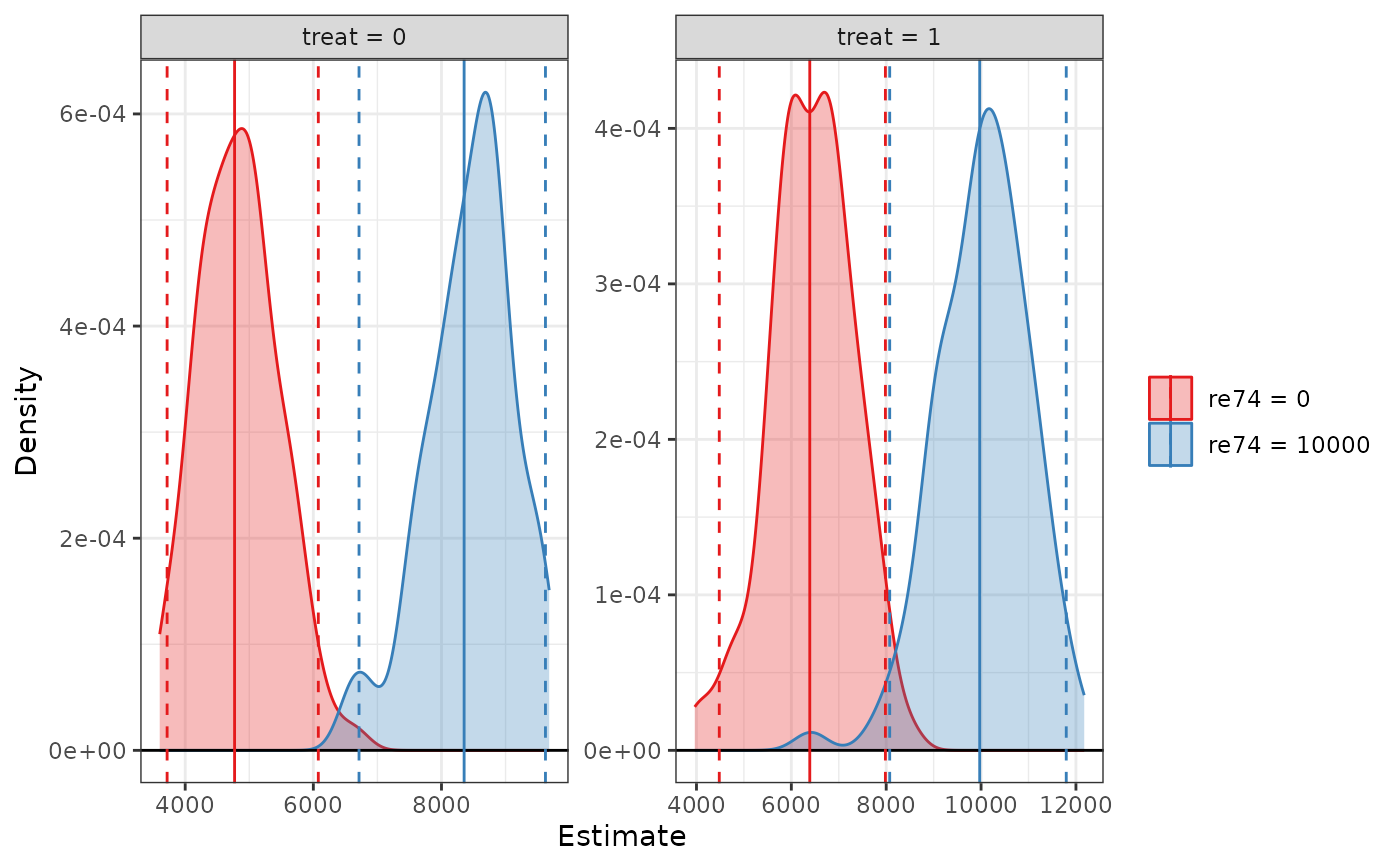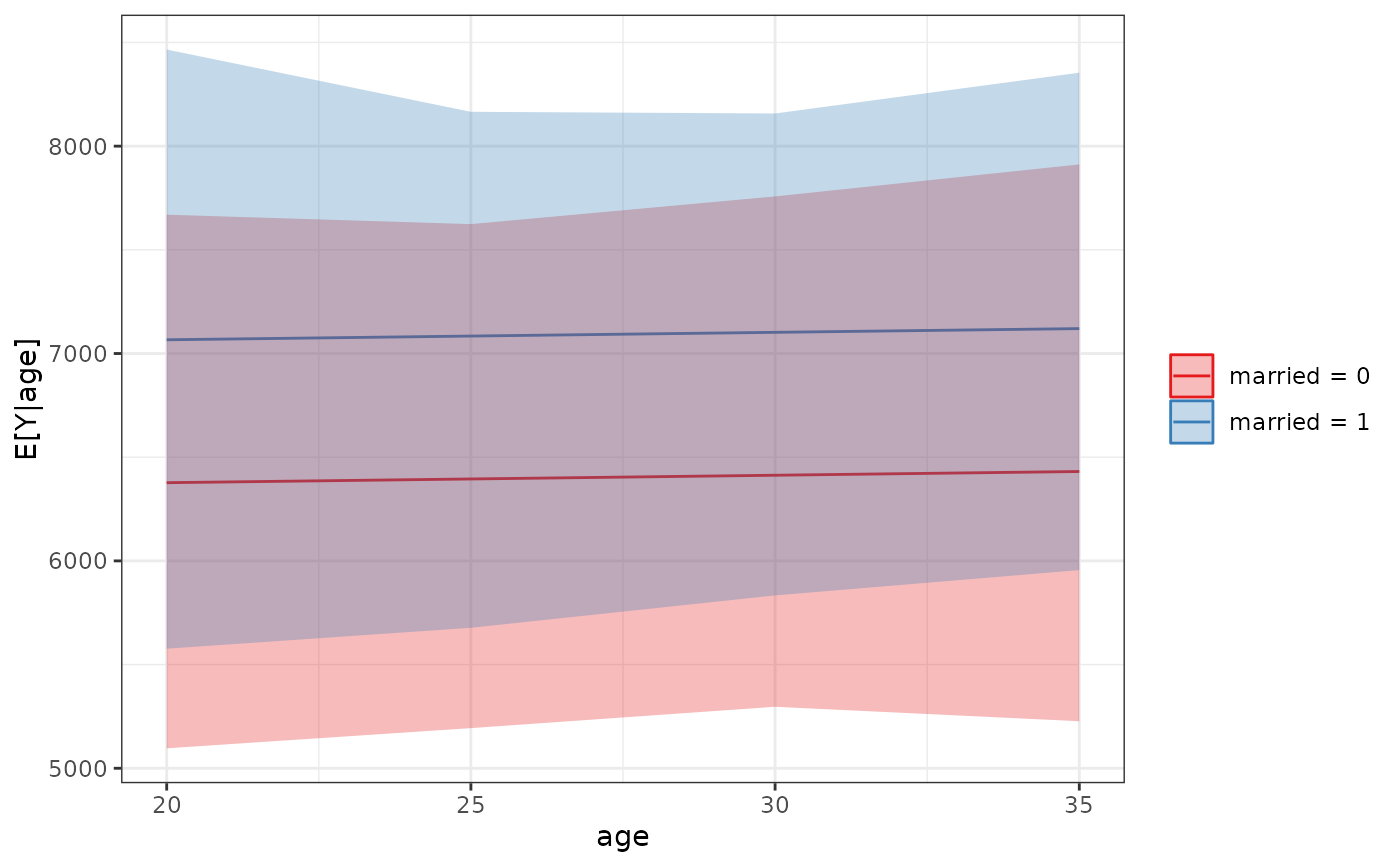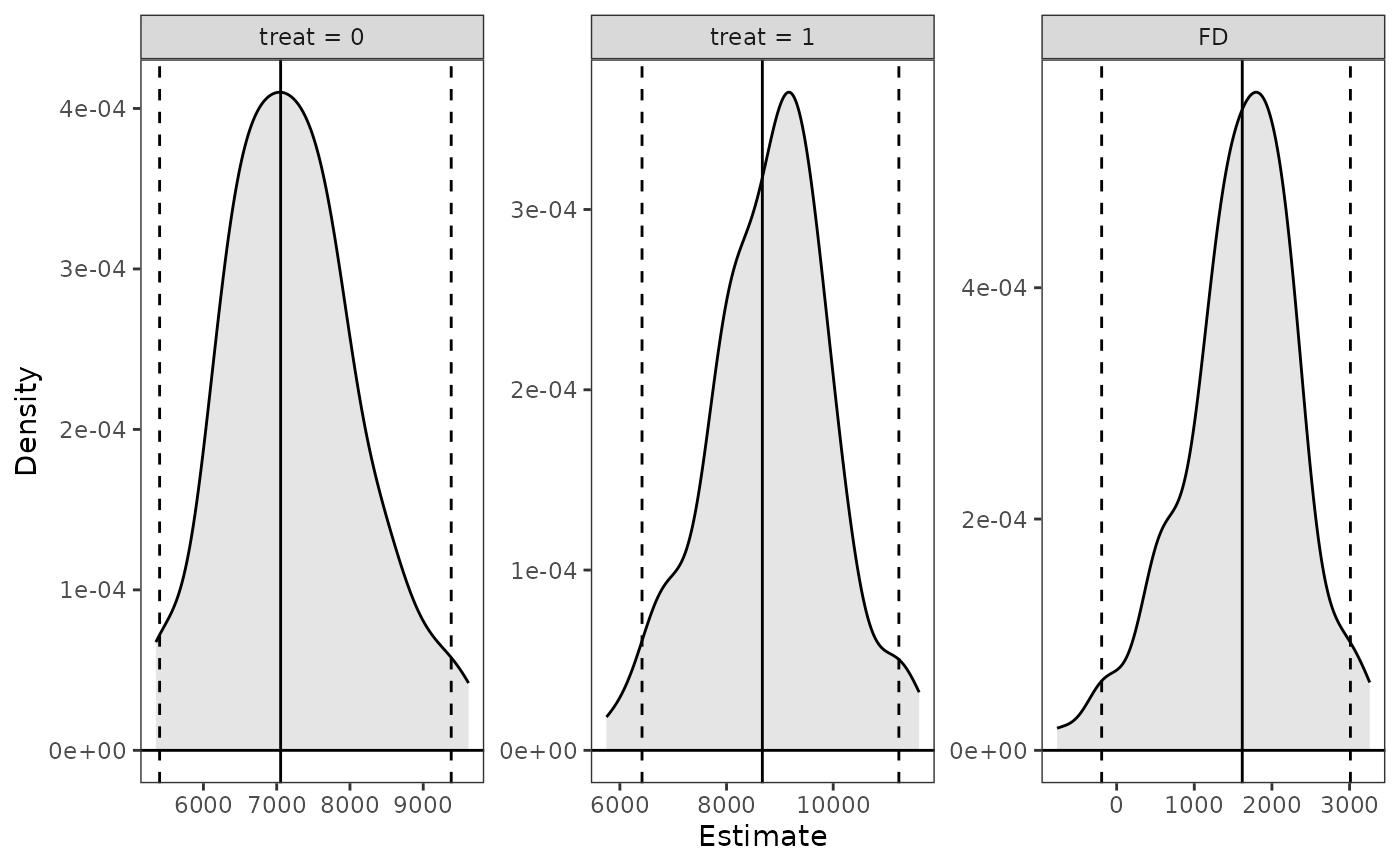sim_setx() is a wrapper for sim_apply() that computes predicted values of the outcome at specified values of the predictors, sometimes called marginal predictions. One can also compute the difference between two marginal predictions (the "first difference"). Although any function that accepted clarify_est objects can be used with sim_setx() output objects, a special plotting function, plot.clarify_setx(), can be used to plot marginal predictions.

## Usage

sim_setx(
sim,
x = list(),
x1 = list(),
outcome = NULL,
type = NULL,
verbose = TRUE,
cl = NULL
)

# S3 method for clarify_setx
print(x, digits = NULL, max.ests = 6, ...)

## Arguments

sim

a clarify_sim object; the output of a call to sim() or misim().

x

a data.frame containing a reference grid of predictor values or a named list of values each predictor should take defining such a reference grid, e.g., list(v1 = 1:4, v2 = c("A", "B")). Any omitted predictors are fixed at a "typical" value. See Details. When x1 is specified, x should identify a single reference unit.

For print(), a clarify_setx object.

x1

a data.frame or named list of the value each predictor should take to compute the first difference from the predictor combination specified in x. x1 can only identify a single unit. See Details.

outcome

a string containing the name of the outcome or outcome level for multivariate (multiple outcomes) or multi-category outcomes. Ignored for univariate (single outcome) and binary outcomes.

type

a string containing the type of predicted values (e.g., the link or the response). Passed to marginaleffects::get_predict() and eventually to predict() in most cases. The default and allowable option depend on the type of model supplied, but almost always corresponds to the response scale (e.g., predicted probabilities for binomial models).

verbose

logical; whether to display a text progress bar indicating progress and estimated time remaining for the procedure. Default is TRUE.

cl

a cluster object created by parallel::makeCluster(), or an integer to indicate the number of child-processes (integer values are ignored on Windows) for parallel evaluations. See pbapply::pblapply() for details. If NULL, no parallelization will take place.

digits

the minimum number of significant digits to be used; passed to print.data.frame().

max.ests

the maximum number of estimates to display.

...

optional arguments passed to FUN.

## Value

a clarify_setx object, which inherits from clarify_est and is similar to the output of sim_apply(), with the following additional attributes:

• "setx" - a data frame containing the values at which predictions are to be made

• "fd" - whether or not the first difference is to be computed; set to TRUE if x1 is specified and FALSE otherwise

## Details

When x is a named list of predictor values, they will be crossed to form a reference grid for the marginal predictions. Any predictors not set in x are assigned their "typical" value, which, for factor, character, logical, and binary variables is the mode, for numeric variables is the mean, and for ordered variables is the median. These values can be seen in the "setx" attribute of the output object. If x is empty, a prediction will be made at a point corresponding to the typical value of every predictor. Estimates are identified (in summary(), etc.) only by the variables that differ across predictions.

When x1 is supplied, the first difference is computed, which here is considered as the difference between two marginal predictions. One marginal prediction must be specified in x and another, ideally with a single predictor changed, specified in x1.

sim_apply(), which provides a general interface to computing any quantities for simulation-based inference; plot.clarify_setx() for plotting the output of a call to sim_setx(); summary.clarify_est() for computing p-values and confidence intervals for the estimated quantities.

## Examples

data("lalonde", package = "MatchIt")

fit <- lm(re78 ~ treat + age + educ + married + race + re74,
data = lalonde)

# Simulate coefficients
set.seed(123)
s <- sim(fit, n = 100)

# Predicted values at specified values of values, typical
# values for other predictors
est <- sim_setx(s, x = list(treat = 0:1,
re74 = c(0, 10000)),
verbose = FALSE)
summary(est)
#>                         Estimate 2.5 % 97.5 %
#> treat = 0, re74 = 0         4771  3645   5841
#> treat = 1, re74 = 0         6389  4653   8147
#> treat = 0, re74 = 10000     8353  7106   9539
#> treat = 1, re74 = 10000     9971  8083  12092
plot(est)# Predicted values at specified grid of values, typical
# values for other predictors
est <- sim_setx(s, x = list(age = c(20, 25, 30, 35),
married = 0:1),
verbose = FALSE)
summary(est)
#>                       Estimate 2.5 % 97.5 %
#> age = 20, married = 0     6377  5314   7439
#> age = 25, married = 0     6395  5273   7424
#> age = 30, married = 0     6413  5216   7555
#> age = 35, married = 0     6431  5136   7700
#> age = 20, married = 1     7066  6000   8512
#> age = 25, married = 1     7084  6164   8266
#> age = 30, married = 1     7102  6249   8077
#> age = 35, married = 1     7120  6122   8226
plot(est)# First differences of treat at specified value of
# race, typical values for other predictors
est <- sim_setx(s, x = data.frame(treat = 0, race = "hispan"),
x1 = data.frame(treat = 1, race = "hispan"),
verbose = FALSE)
summary(est)
#>           Estimate 2.5 % 97.5 %
#> treat = 0     7054  5731   8592
#> treat = 1     8672  6403  11112
#> FD            1618   268   3078
plot(est)